﻿ The Faults Diagnostic Analysis for Analog Circuit Faults Based on Cuckoo Search Algorithm and BP Neural NetworkPublications are Open
Access in this journal
Article Versions
Export Article
• Normal Style
• MLA Style
• APA Style
• Chicago Style
Research Article
Open Access Peer-reviewed

### The Faults Diagnostic Analysis for Analog Circuit Faults Based on Cuckoo Search Algorithm and BP Neural Network

LingZhi Yi, Yue Liu , WenXin Yu, Weihong Xiao
American Journal of Electrical and Electronic Engineering. 2017, 5(3), 102-107. DOI: 10.12691/ajeee-5-3-5
Published online: June 02, 2017

### Abstract

Neural networks have many advantages, such as parallel processing, self-suit, associated memory and classify ability strongly which can be used to analog circuit fault diagnosis. But it is very easy to trap the local minimum if the initial network weights are randomly generated. To solve this problem, the cuckoo search algorithm is used to optimize the initial weights of the neural network. A novel method for analog circuit fault diagnosis is proposed in this paper, based on BP neural network as classifier optimized by cuckoo search algorithm. The feasibility and effectiveness of the proposed method are verified by the simulations of Sallen-Key low-pass filter circuit. Compared with other methods, the results show that the proposed method is effective to identify and classify faults.

### 1. Introduction

Since the 1960s, analog circuit fault diagnosis has made a lot of achievements, scholars have put forward many methods, such as Fault Dictionary, Statistical Identification, Gray Recognition method 1. The traditional dictionary diagnosis is the most effective method for the fault diagnosis of analog circuit currently, but it needs much works before the simulation and if the inputs don’t match the reserved values accurately, the system will fail to diagnosis 2. In fact, the voltage and current on the test spot can’t completely match the reserved values in the dictionary, which will result in failure inevitably. With the development of the fault diagnosis of analog circuit and the circuit complication and integration continuously increase, the problems of fault diagnosis become more complex and difficult 3.

The fault diagnosis based on artificial intelligence technique is widely used because the traditional one can’t satisfy the demand 4. BP Neural network 5 has strong nonlinear mapping ability, dealing with complex nonlinear problems well, it become the first to use for analog circuit fault diagnosis and has been widely used. The fundamental thought is converting the network using some representation sample to a general dictionary, and the diagnostic information is included in the network’ weigh 6. In this way, if you input the scale characteristic of the circuit, the diagnosis will be found out.

However, the traditional neural network has the shortcomings of its gradient descent search algorithm, it is very easy to trap the local minimum if the initial network weights are randomly generated 7. Moreover, the learning speed of BP network is very slow, and it usually needs thousands of iterations to converge to the global minimum. Cuckoo search algorithm, as a new meta-heuristic optimization algorithm 8, is an efficient approach to solve continuous function optimization problems. In this paper, the neural network will be optimized by cuckoo search algorithm to get improved classification performance for fault diagnosis. The example of Sallen-Key low-pass filter circuit shows that the algorithm classification holds high classified accuracy.

The rest of this paper is organized as follows: Section II introduces the algorithms of cuckoo search and the BP neural network structure in fault diagnosis. Section III analyzes the fault diagnosis of analog circuit based on CS-BP. Section IV shows the simulation and results. Finally, summarize the whole paper in section V.

### 2. CS AND BP

In this section, the theoretical knowledge of the cuckoo search algorithm and neural network are developed.

2.1. Cuckoo Search Algorithm (CS)

Cuckoo search algorithm, as a comparatively new meta-heuristic algorithm, is one of the population based methods developed by Yang and Deb in 2009 9. In the natural, the way of cuckoo breeding is very special. They lay their eggs in the nest of host birds for other species and use host birds’ nest to hatch their eggs and bring up their chicks. A special case of random walk with heavy-tailed probability distribution can be described by the mathematical formulation of levy flight. Cuckoos usually set their eggs in the nests where host birds just laid their eggs. Unfortunately, if host birds discover the eggs which are not their own, they will throw the alien eggs away or abandon their nests and rebuild new nests.

Basic definitions can be summed up as following:

Definition 1: The update of the birds nest positions is: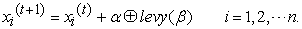(1)

Where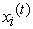denotes the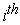cuckoo for the birds' nests in theiteration,means walking randomly by levy flight, where denotes the step size, which is related to the sizes of the problem of interest, and the product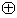denotes entry-wise multiplications.

Definition 2: A levy flight is a random walk in which the step-lengths are drawn from a levy distribution as follow:(2)

Definition 3: In Mantegna’s algorithm, the step lengthcan be calculated: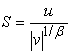(3)

Whereis a parameter between [0, 1] interval and considered to be 1.5;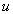and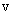are drawn from normal distribution as: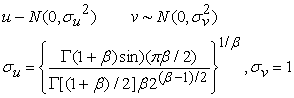(4)

Where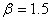in most literature.

Definition 4: The local random work is carried out as: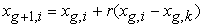(5)

Whereandare two different solutions selected randomly by random permutation. r is random numbers distributed in [0,1]. The basic steps of CS can be summarized as the following:

Step 1: Initialize the parameters of the algorithm. Such as the number of nests n, the Max Generation T, the current iteration t, and the value of.is the detection probability.

Step 2: Generate an initial population of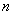host nests regarded as the potential optimal parameter.

Step 3: Calculate the fitness function corresponding to cuckoo algorithm, and find the current best.

Step 4: Update the nest location according to the location update formula of the CS algorithm by (1), the new nest location can be get and take them back to step 3. Get the fitness value, compared with the last generation. Get the current optimal position of bird's nest replace the poor bird's nest.

Step 5: If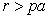, it means that host bird has found cuckoo’s egg and remove them from nest that cuckoo have to search for another place for laying egg, which has been shaping a new generation . Finally, the optimal is chosen to compare with their fitness value.

Step 6: If the number of iterations is equal to the Max Generation, break to step 7; else, the iteration number plus one, back to step 4.

Step 7: Save the best solution

2.2. BP Neural Network Structure in Fault Diagnosis

With the characteristics of simple structure, easy to achieve and robust, BP network is widely used in fault diagnosis. The theoretical research shows that the three-tier BP network, as long as the network structure and parameter selection is reasonable, accuracy will approximate any nonlinear functions. The first is to extract the fault feature, which is the key to fault diagnosis, but also the basis for constructing the sample set. The nodes are measured in the circuit, and then the data is preprocessed and the scale is changed to form the set of fault feature samples. It is divided into training sample set and test sample set. The extracted fault feature sample set is used as the input of the neural network. The network output is both the membership degree of the fault, the expected output is binary code, the value of the fault is 1 and the rest is 0.

Constructing neural networks as shown in Figure 1, the output of the network element is:

• Figure 1. The structure of Neural Network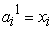(6)(7)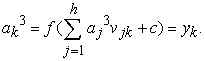(8)

Theunit of the input layer for the BP network is,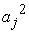is the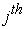unit of the hidden layer,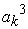is theunit of the output layer,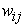is the connection weight of theunit of the input layer tounit of the hidden layer, Similarly,is the connection weight of theunit of the hidden layer to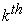unit of the output layer.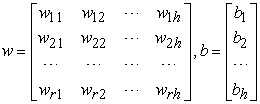(9)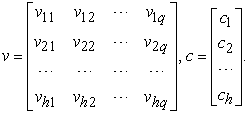(10)

The network output is compared with the expected output, calculate the network error, if satisfy the required error that stop the training, in contrast to change the network weight, cycle training straight to meet the requirements.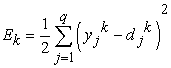(11)(12)(13)(14)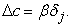(15)

In the above formula,,and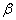is the learning rate.

### 3. Fault Diagnosis of Analog Circuit Based on CS-NN

In this section, the proposed method will be described for fault diagnosis of analog circuit.

The fault detecting and orientation of analog circuit are similarly to classify issues, and the neural network has the classify ability strongly which can be used to fault diagnosis. The cuckoo search algorithm is used to optimize the BP network. In the optimization process, each cuckoo individual represents a weight and threshold combination, the merits of each individual depends on the fitness function. The cuckoo search algorithm finds the individuals corresponding to the optimal fitness value by performing the CS optimization process. Anti-coded the optimal cuckoo individual by the CS algorithm, and the optimal combination of weights and thresholds are obtained.

When one component is in a fault, the amplitude-frequency curve will have certain changes. The characteristics of fault data can be extracted by it. In Figure 1, the algorithm schematic of CS combined with BP is given for analog circuit fault diagnosis. Drive signal is regarded as input of analog circuit, fault information is regarded as output of that. Then samples will be normalized, and training data and testing data will be sorted. Note that the training and testing samples may have certain correlations, so principal component analysis (PCA) is necessary to eliminate redundancy information and reduce complexity. The parameters w and B of BP network will be optimized by CS algorithm, then the classified results could be obtained after test samples are put into optimal classified model with cross validation.

Figure 2 is the flow chart of analog circuit faults diagnosis, followed by data normalization, feature extraction and principal component analysis parameter optimization of BP by CS, CS-BP fault diagnosis.

• Figure 2. The flow chart of analog circuit faults diagnosis

The processes of our proposed method is as followed in the Figure 3, classify the analog circuit faults with the trained BP network classifier.

• Figure 3. The processes of BP classifier optimized by Cuckoo Search Algorithm

The processes of BP classifier optimized by Cuckoo Search Algorithm in our proposed method is as followed:

Initialization:

Step 1: Initialize the parameters of the algorithm, such as the number of nests n, the Max Generation T, the current iteration t, and the value of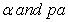.

Step 2: Construct BP classified model and set the neuron number and the search space in each layer.

Search process:

Step 3: Generate an initial population of n host nests regarded as the potential weight and threshold combinations.

Step 4: Calculate the fitness function corresponding to cuckoo algorithm, and find the current best. The fitness value is the sum of absolute errors between the classified value and the actual value.

Step 5: Update the nest location according to the location update formula of the CS algorithm by (1), we can get the new nest location and take them back to step 4. Get the fitness value, compared with the last generation. Get the current optimal position of bird's nest replace the poor bird's nest.

Step 6: If, it means that host bird has found cuckoo’s egg and remove them from nest that cuckoo have to search for another place for laying egg, which has been shaping a new generation. Finally, the optimal is chosen to compare with their fitness value.

Step 7: If the number of iterations is equal to the Max Generation, break to step 8; else, the iteration number plus one, back to step 5.

Classification:

Step 8: Get the best weight and threshold combinations, and construct the classified model. Using the training set to train the network, when the training error is no longer reduced, stop training.

Step 9: Classify the analog circuit faults with the trained BP classifier.

### 4. The Simulation and Results

In this section, the Sallen-Key low-pass filter circuit will be tested to verify the feasibility and effectiveness.

4.1. The Circuit under Test and Parameters Settings

The normal value of every component is shown in Table 1. And resistors and capacitors have 5% tolerance, respectively. Every normal value is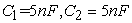. Here we assume resistors and capacitors in this interval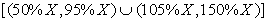(X is the normal value). Then faults can be classified to 8 fault models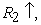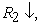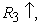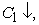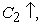Parameters of diagnostic classification method are defined as following: the input number is 4, the hidden number is 8 and the output number is 9 in the BP network. The sizes of cuckoos and the number of iteration are all 25.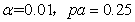.

Where the meaning of ‘↑’ is that value of component in fault comes to larger than normal value. Likewise, the ‘↓’ is that value of components in fault comes to smaller than standard. And all patterns have 9 kinds of fault styles, including trouble-free style. The diagnosis Sallen-Key low-pass filter circuit, as shown in Figure 4, is simulated by a circuit simulation software OrCAD10.5. The circuit is driven using sinusoidal voltage signal of 5V, and the corresponding frequency-voltage value of fault feature could be got according to the output amplitude- frequency curve. Finally, 20 features, 720 samples of 9 categories could be got by Monte Carlo Analysis.

• Figure 4. Sallen-Key low-pass filter
4.2. Discussion and Analysis

From the point of view of classifier’s performance, the desirable results have been obtained in a few iterations with high classification rates. Figure 5 shows the classified results of classified data and actual data, which only one data couldn’t classified correctly. Even classified date all equal the actual date, we can see it can also be completely diagnosed as shown in Figure 6. Table 2 shows the detailed results of our proposed method to diagnose 8 fault styles with Sallen-Key low-pass filter. Every row corresponds to one fault style in this table. Different grid indicates the classified fault data belonging to various fault styles. For instance, All 20 test data of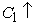was correctly classified in the first row and first column. However, that only 19 test data has been diagnosed correctly in the third row and another one is incorrectly diagnosed to NF (normal state) in the ninth row and third column. In Figure 7, we can see the best validation performance is 0.010903 at epoch 53, and the mean squared error (MSE) is gradually descend and then stabilize.

• Figure 5. Curves of the actual and classified data
• Figure 6. Curves of the actual and classified data
• Figure 7. Curves of the mean squared error

There are many methods that have been proposed for fault diagnosis for Sallen-Key low-pass filter in the last few years. Now, we make comparison and discussion between our proposed method and others. A novel strategy for fault diagnosis of analog circuit online based modified kernel fuzzy C-means was proposed in 10, the accuracy can be reached 95%. Ref. 11 proposed analog fault diagnostic method based on wavelet neural networks (WNN) for actual circuits. And genetic algorithm was presented to optimize the structure and the parameters of WNN in the training process. However, the accuracy rate (99.44%) of our method is obviously higher than their accuracy rate (95%). The faults diagnostic analysis for analog circuit faults based on firefly algorithm and extreme learning machine was proposed in ref. 12, the proposed method takes advantages of excellent classification capacity of extreme learning machine. But, their accuracy rate is 97.775%, which is lower than our method (99.44%). Table 3 shows the comparisons between proposed method and others.

### 5. Conclusions

In this paper, a novel method for analog circuit fault diagnosis based on BP neural network as classifier optimized by Cuckoo Search Algorithm is proposed. The proposed method takes advantages of excellent classification capacity of BP neural network, and a good trade-off between exploration and exploitation of cuckoo search algorithm. The best weights and bias value would be optimized by cuckoo search algorithm in order to improve the fault classification accuracy. The Sallen-Key low-pass filter has been simulated as the circuit under test. The simulation and comparison results show that this method has better performances and is indeed more effective and reliable than other methods proposed in past literatures.

### Acknowledgements

This work was supported by the National Natural Science Foundation of China(61572416), Hunan Provincial Natural science Zhuzhou United foundation (2016JJ5033), JCYJ2016042911221382), Key discipline of Hunan Province ‘Information and Communication Engineering’ in ‘12th Five Year Plan’. HuNan University of Science and Technology Doctor Startup Fund Grant No.E51664. Hunan Provincial Department of Education Science Research Project Grant No.16C0639.

### References

  Shen Y, Ding S X, Haghani A, et al. A comparison study of basic data-driven fault diagnosis and process monitoring methods on the benchmark Tennessee Eastman process. Journal of Process Control, 2012, 22(9): 1567-1581. In article View Article  Henckels L, Haas R, Anderson R. Method of and apparatus for automatic fault diagnosis of electrical circuits employing on-line simulation of faults in such circuits during diagnosis: US, US 4228537 A. 1980. Bao Y, Guo Z. Study of Circuit Fault Diagnosis Method Based on BP Algorithm. Electronic Science & Technology, 2016. In article View Article  Gui W H, Liu X Y. Fault Diagnosis Technologies Based on Artificial Intelligence for Complex Process. Basic Automation, 2002. In article View Article  Mohammadi K, Monfared A R M, Nejad A M. Fault diagnosis of analog circuits with tolerances by using RBF and BP neural networks/ Research and Development, 2002. SCOReD 2002. Student Conference on. IEEE, 2002:317-321. In article View Article  Moody J O, Antsaklis P J. The dependence identification neural network construction algorithm [J]. IEEE Transactions on Neural Networks, 1996, 7(1):3-15. In article View Article  PubMed  Sadeghi B H M. A BP-neural network predictor model for plastic injection molding process. Journal of Materials Processing Technology, 2000, 103(3): 411-416. In article View Article  Gandomi A H, Yang X S, Alavi A H. Cuckoo search algorithm: a metaheuristic approach to solve structural optimization problems. Engineering with Computers, 2013, 29(1): 17-35. In article View Article  Valian E, Valian E. A cuckoo search algorithm by Lévy flights for solving reliability redundancy allocation problems. Engineering Optimization, 2013, 45(11):1273-1286. In article View Article  Yang X S. Metaheuristic optimization: Nature-inspired algorithms and applications. Studies in Computational Intelligence, 2013, 427:405-420. In article View Article  Zhang Z, Zhang A. A novel strategy for fault diagnosis of analog circuit online based modified kernel fuzzy C-means// IEEE International Conference on Industrial Technology. IEEE, 2016:938-943. In article View Article  S. Guoming, W. Houjun, J. Shuyan, and L. Hong, “Fault diagnosis approach of analog circuits based on genetic wavelet neural network,” ICEMI '07. 8th International Conference on Electronic Measurement and Instruments, 2007, pp. 3-675-3-679. In article View Article  Yu W X, Sui Y, Wang J. The Faults Diagnostic Analysis for Analog Circuit Based on FA-TM-ELM[J]. Journal of Electronic Testing, 2016(4):1-7. In article View ArticleThis work is licensed under a Creative Commons Attribution 4.0 International License. To view a copy of this license, visit http://creativecommons.org/licenses/by/4.0/